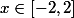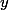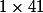# Martin Orr's Blog

## Matlab rant: Elementwise operations on arrays

Posted by Martin Orr on Saturday, 17 March 2012 at 17:27

I have to teach some simple use of Matlab to my engineering students. I don't much like the Matlab language, but then I am unhappy with pretty much every programming language I have ever used except C (which is great for some purposes but they do not include numerical work). Today I am going to rant about the first bit of code in our Matlab course (and probably the first non-trivial bit of code in many Matlab courses and tutorials).

My complaint concerns elementwise operations on arrays (i.e. applying the same operation to every element of an array). These are very important in Matlab, and sometimes you can write code that looks like it is acting on scalars and it just works elementwise on arrays. If scalar code would always just work elementwise on arrays then I might be happy with this (but I worry that if you had such a language, how would you distinguish operations that act on an array as a unit, not just elementwise?).

But sometimes you need to alter things slightly to work elementwise on arrays. I worry that for beginning programmers, the fact that elementwise array operations look so similar to scalar operations makes it difficult to understand when these alterations are required - it would be better to consistently require a syntax meaning "perform this operation elementwise."

### Elementwise multiplication

Consider the following piece of code, which is the first example in my Matlab course.

    x = -2 : 0.1 : 2;
y = x .* x;
plot(x, y);


This displays a graph of the functionfor. In more detail:

1. x = -2 : 0.1 : 2 creates an array x containing the 41 elements. The teaching material I have calls this a vector rather than an array. I am not sure if that is easier terminology or not; the fact that, for Matlab, arrays and vectors are the same is crucial at one point later on.

2. y = x .* x multiplies each element of x by itself, putting the result in a new array y.

3. plot(x, y) plots a graph of the points withcoordinates given by the first argument andcoordinates given by the second argument, then joins them up with straight line segments.

The key issue for us is the . before the * in the second line. This indicates that we want to apply the multiplication operation elementwise. So this is one of those situations where you have to alter the scalar code slightly to work elementwise on arrays.

If you leave out the dot, which is a common mistake for beginners, then you get a hard-to-understand error message:

    x = -2 : 0.1 : 2;
y = x * x;

??? Error using ==> *
Inner matrix dimensions must agree.


Without the dot, * means matrix multiplication. Our 41-element array x is interpreted as amatrix, which cannot be multiplied by itself (because matrix multiplication is only defined if the number of columns of the first matrix is equal to the number of rows of the second). Since my students do not yet know about matrix multiplication, it is hard to explain this to them.

I think it would be a simple but major improvement to Matlab if it offered the suggestion “Perhaps you meant .*" as well as the error message.

The same thing happens with division as with multiplication; indeed it is worse because there is no mysterious error message, just a mysteriously incorrect graph at the end.

On the other hand, to add arrays, you must use + without a dot. It makes sense that + should be allowed because vector addition is defined to be elementwise addition, but I think that .+ should be allowed as a synonym.

### Elementwise functions

If we wanted to plot a graph of the sine function, we would replace the second line of code by

    y = sin(x);


This looks identical to code operating on a scalar, but in fact it computes the sine of each element of the array. It seems to me that consistency requires that you should have to use a dot to indicate that you want to apply the sine function elementwise:

    y = sin.(x);


My students do not seem to find anything troubling in the elementwise use of sin that we see here, but it causes trouble when they come to write their own functions.

The problem lies in the fact that the above code does not, as you might think, call sin repeatedly with scalar arguments: it calls sin once with an array argument. It is part of the definition of the built-in function sin that when called with an array argument, it operates on each element of the array.

So if you write your own function and want to call it in the same way, you have to take care when writing the function that it can accept vector arguments. For example the following does not work:

    function u = f(t)
u = t * t;
end

x = -2 : 0.1 : 2;
y = f(x);


In this simple case you have to use .* in the function definition. In more complicated cases you might have to write a loop inside the function.

On the other hand my proposed syntax of adding a dot before the parenthesis would work with any function taking a scalar argument.

### Variable names

This is not a complaint against the language but rather about the example code I have been using. One potential source of confusion is that we have used x and y to mean not scalar values but rather arrays whose elements are what we really think of as values ofand. Perhaps it would help to entangle some of the above issues if we called them xs and ys instead:

    xs = -2 : 0.1 : 2;
ys = xs .* xs;
plot(xs, ys);


This naming convention is common in the functional programming world. If you have not seen it before I should perhaps explain that you read xs as the plural of x.